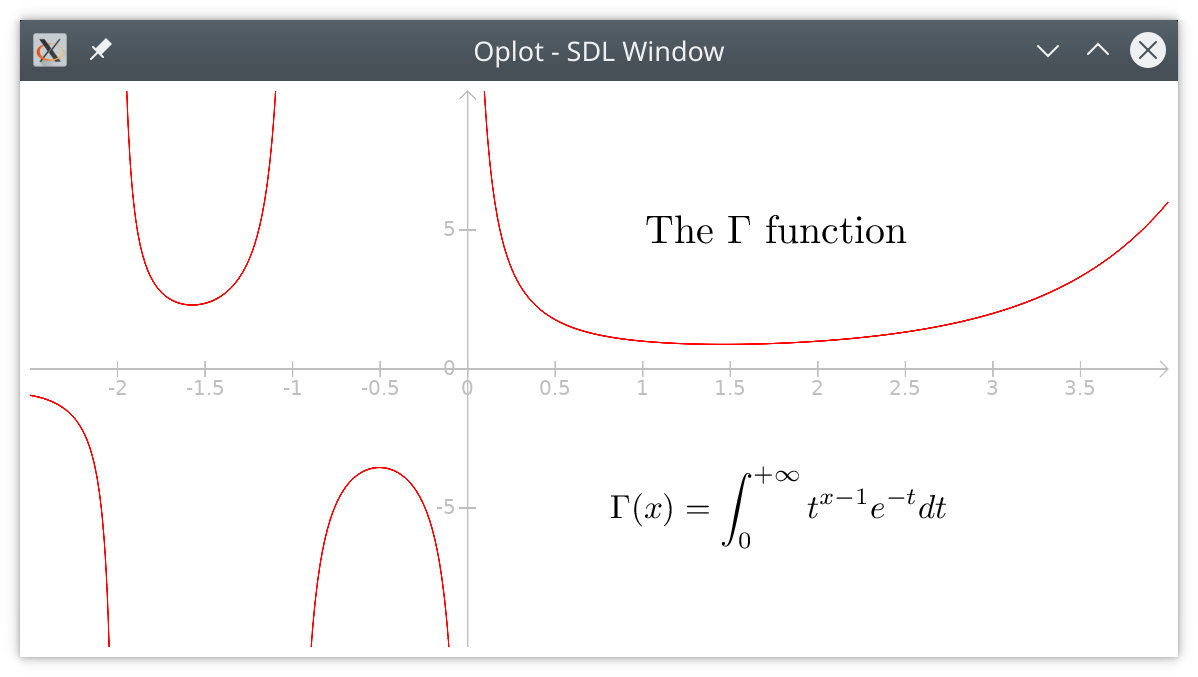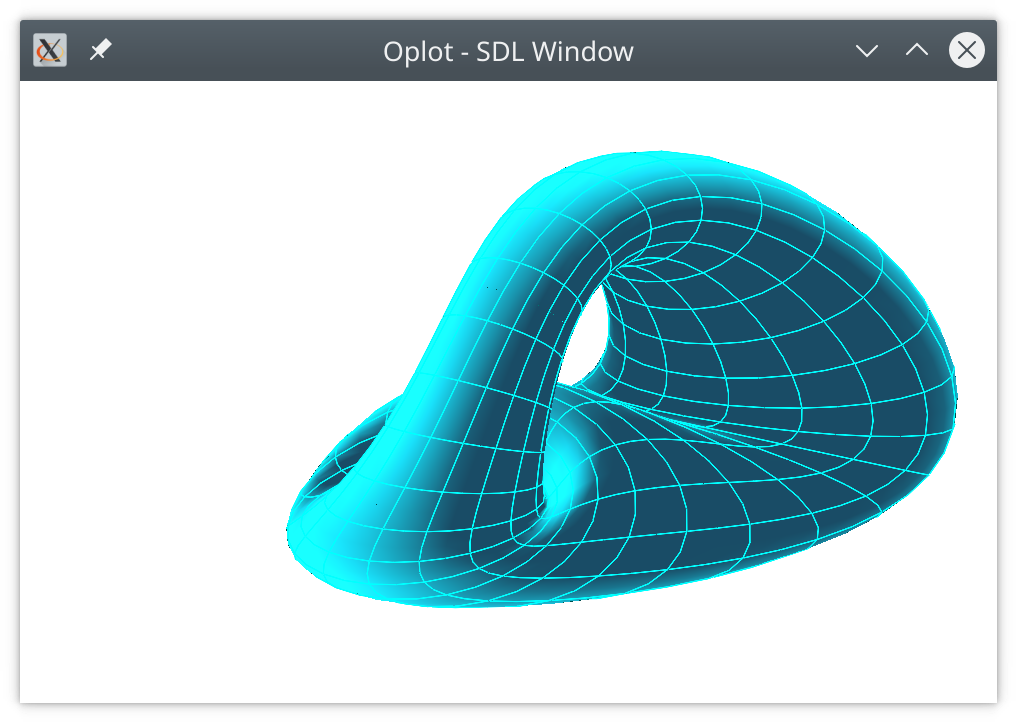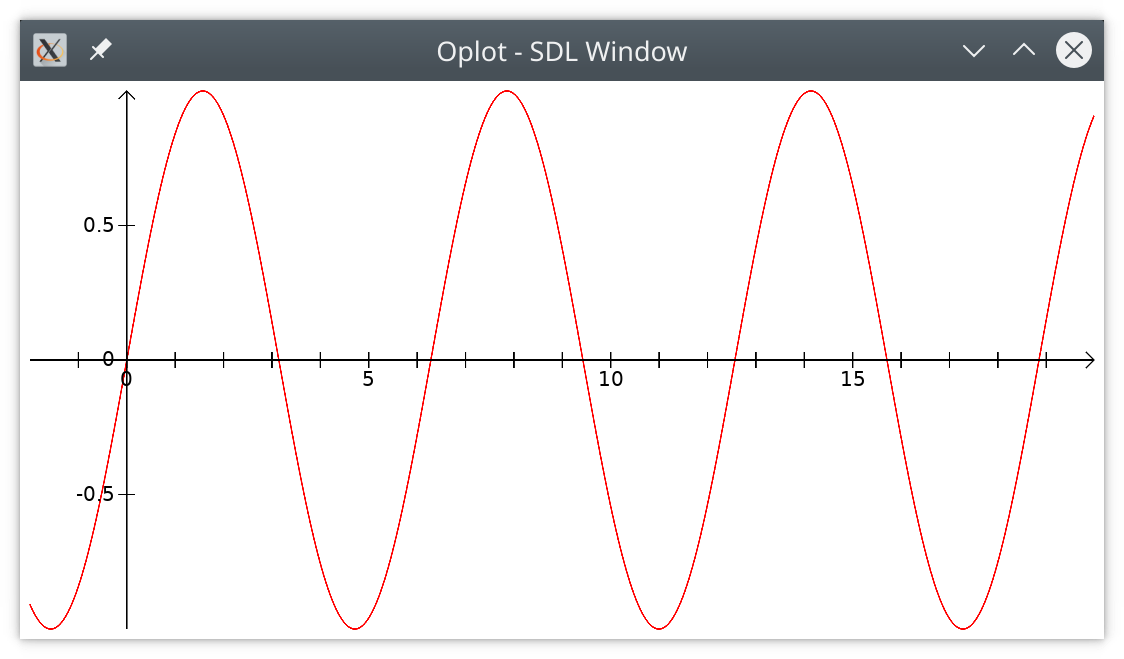# Module `Oplot`

Simple mathematical plotter library for `ocaml` with fast graphics (opengl), LaTeX display, and high quality vector output (xfig, postscript or PDF)Source available on github.

• version 0.71

## Example

`Oplot` can be used in the toplevel. First load the library with

``````#use "topfind"

#require "oplot"``````

You may open the `Oplot.Plt` module for easy access to all plot functions.

``open Oplot.Plt``

Draw the graph of the `sine` function with

``````let p = plot sin (-2.) 20.
let a = axis 0. 0.;;

display [ Color red; p; Color black; a ]``````

This will open a window with the graphics, which should look like this:Press `F` for fullscreen toggle, `CTRL-S` for saving the image, and `ESC` or `Q` to close the window. Press `h` to see the list of active keys.

Of course you can play with it:

``````let rec sh i =
if i == 0 then []
else
let p =
line_plot_f
(fun x -> sin (x +. (float_of_int i /. 50.)))
0. 20. ~step:0.05
in
let c =
color (float_of_int i /. 50.) (1. -. (float_of_int i /. 50.)) 0.
in
c :: p :: sh (i - 1)
;;

display (sh 50)```````module Points : sig ... end`

Types of points

## Main Oplot functions

This module contains all plotting functions.

`module Plt : sig ... end`